Courses

# Expectation and moments - Probability and probability Distributions, CSIR-NET Mathematical Sciences Mathematics Notes | EduRev

## Mathematics : Expectation and moments - Probability and probability Distributions, CSIR-NET Mathematical Sciences Mathematics Notes | EduRev

The document Expectation and moments - Probability and probability Distributions, CSIR-NET Mathematical Sciences Mathematics Notes | EduRev is a part of the Mathematics Course Mathematics for IIT JAM, CSIR NET, UGC NET.
All you need of Mathematics at this link: Mathematics

1. Introduction

Functions of random variable are discussed in previous lectures. In this lecture, properties of random variable, e.g. expectation, moments and moment-generating function of ‘functions of random variable’ are discussed in detail.

2. Usefulness of the properties of random variables

The properties of random variables are useful for statistical problems in civil engineering. Standard procedure can be followed to obtain the moments and expectations from the probability distribution functions, as described in previous lectures. There are different methods to obtain pdf of the functions of random variable available, though the procedure might be complex in few cases. For such instances, information of moments of the derived random variables is very useful.

3. Expectation of functions of discrete random variables

Expectation of the discrete random variable X is given by,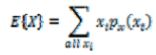Therefore, the expectation of the function of a discrete random variable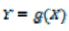is expressed as,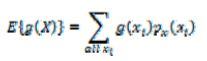Expectation of the continuous random variable X is given by,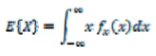3.1. Properties of Expectation

The following properties of expectation hold good.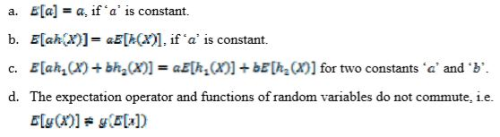3.2. Example for discrete random variables
Problem 1. The random variable  has a probability mass function (pmf)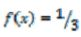for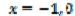and . Find the mean of the function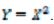Solution.  The mean of the function,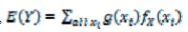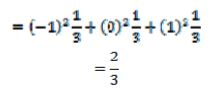3.3. Example for discrete random variables

Problem 2.
Assume that the inter-arrival time, x of a vehicle approaching a toll station of a bridge has an exponential pdf with parameter λ  . There are k toll lines in that toll station. Thus k vehicles can be accommodated at a time. Determine the mean arrival time of  k vehicles and the coefficient of variation of this arrival time. Assume that the arrivals are independent of each other (Kottegoda and Rosso, 2008).

Solution.  As the inter-arrival time,  follows an exponential distribution, the mean and the variance of  are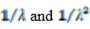respectively.
The total time for the arrival of  k vehicle is denoted as . Thus we get,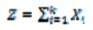where, Xis the arrival time of the i th vehicle

and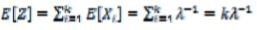Hence the coefficient of variation is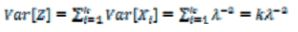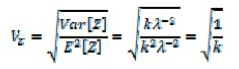Thus the variation of arrival time decreases with increase in toll lines, as ‘ ’ is a positive real number.

4. Moments of functions of random variables

In general, the rth moment of the function of discrete random variable g(x) is given by: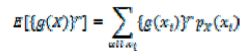And the rth moment of the function of continuous random variable g(x) is given by: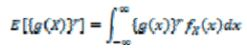4.1. Moments of functions of random variables about its mean

The rth moment of the function of discrete random variable Y = g(x) about its mean is given by: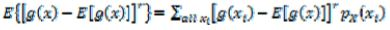The rth moment of the functions of continuous functions Y = g(x) is expressed as: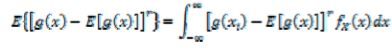4.2. Variance of discrete functions

The variance of discrete function, Y = g(x) is expressed as: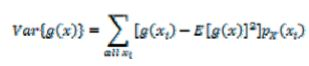4.3. Variance of continuous functions

The variance of discrete function, Y = g(x) is expressed as: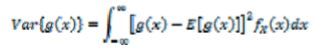5. Mean and Variance of Linear Function

Let us consider a linear function as,  Y = aX + b, where a and b are constants.

The mean values of Y  is mathematical expectation of aX + b, i.e.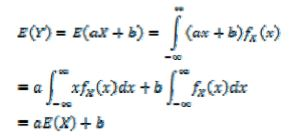Similarly, variance of Y can be expressed as,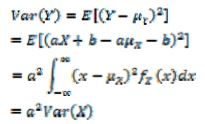6. Expansion of Functions of Random Variable

The function of random variable, g(x)  can be expanded in a Taylor series about the mean value, μr.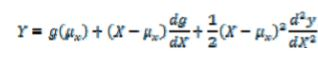where derivatives are evaluated at .
If the series is truncated at linear terms, then the first-order approximate mean and variance of Y are obtained.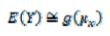The variance of function of random variable,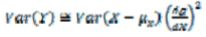It should be noted that, if the function, g(x) is approximately linear for the entire range of value , then above two equations will yield good approximation of exact moments.

Problem 3. The length of two rods will be determined by two measurements with an unbiased instruments with an unbiased instrument that make random error with mean 0 and standard deviation σ in each measurement. Compute the variance in the estimation of the length Tand T2 by the following methods:
a. The two rods are measured separately
b. The sum and difference of the length of two rods are measured instead of individual lengths (Ang and Tang, 1975)

Solution. a. Let  and  denote the measurement obtained for the two rods, then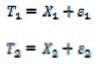where, s1, s2 are the errors involved in the measurements.
The variance in the estimation of T1  is: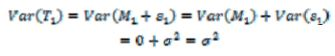Similarly,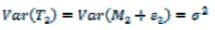b. Suppose M3 denotes that the measured combined length of the two rods and  Mdenotes the measured difference between the lengths of the two rods. Then,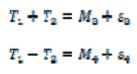Solving these two equations, we get,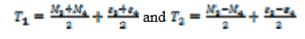Assuming that the errors are statically independent, the variance in the estimation of T1 is therefore,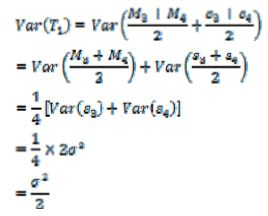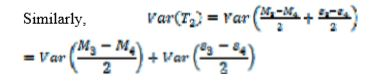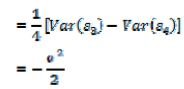From this, we can further find that the second method of measuring the length of the rods is better, since the variance in the estimation of the true lengths T1 and T2, are smaller.

Offer running on EduRev: Apply code STAYHOME200 to get INR 200 off on our premium plan EduRev Infinity!

197 docs

,

,

,

,

,

,

,

,

,

,

,

,

,

,

,

,

,

,

,

,

,

,

,

,

;